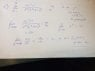# Did I apply this limit comparison test correctly?

• Randall
This is not true, and is a common mistake. I suggest that you go back and try to correct those mistakes.f

## Homework Statement

Use the limit comparison test to show the series converges or diverges: Sum from n=1 to infinity of ((5n^3)+1)/((2^n)((n^3)+n+1))

## Homework Equations

suppose Sum An and Sum Bn are two positive series. Let lim as n goes to infinity of An/Bn = c: 1) if 0<c<inifinity then either both converge or both diverge; 2) if c=0 and Sum Bn converges, so does Sum An; 3) if c=infinity and Sum Bn diverges, so does Sum An

## The Attempt at a Solution

See attached - series An is very complex. I'm not sure it goes to infinity like I assumed, nor am I sure that I picked an appropriate Bn equation. Please help thanks.

#### Attachments

•limit_comparison_test_2.jpg
31.6 KB · Views: 398

## Homework Statement

Use the limit comparison test to show the series converges or diverges: Sum from n=1 to infinity of ((5n^3)+1)/((2^n)((n^3)+n+1))

## Homework Equations

suppose Sum An and Sum Bn are two positive series. Let lim as n goes to infinity of An/Bn = c: 1) if 0<c<inifinity then either both converge or both diverge; 2) if c=0 and Sum Bn converges, so does Sum An; 3) if c=infinity and Sum Bn diverges, so does Sum An

## The Attempt at a Solution

See attached - series An is very complex. I'm not sure it goes to infinity like I assumed, nor am I sure that I picked an appropriate Bn equation. Please help thanks.

I wish you would take the trouble to type out the answer, rather than giving it in a rather messy screen shot. (For more on this issue, see the pinned posting "Guidelines for Students and Helpers", by Vela.) However, as far as I can see, what you wrote is not in any way relevant to the problem. However, I won't say more now, since I think you need to go back and think carefully about what you are doing.

Last edited by a moderator:
I wish you would take the trouble to type out the answer, rather than giving it in a rather messy screen shot.
I agree. In addition to what Ray said, posting an image of your work rather than a typed version of your work prevents us from pointing out exactly where your mistake is.

I am closing this thread. For tips on how to use LaTeX to format mathematics expressions, see https://www.physicsforums.com/help/latexhelp/.

Ray Vickson said:
(For more on this issue, see the pinned posting "Guidelines for Students and Helpers", by Vela.) However, as far as I can see, what you wrote is not in any way relevant to the problem. However, I won't say more now, since I think you need to go back and think carefully about what you are doing.
I see several mistakes in your work, one of which is saying that ∞/∞ = ∞.